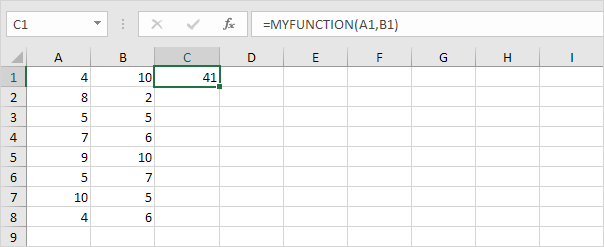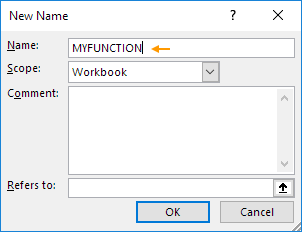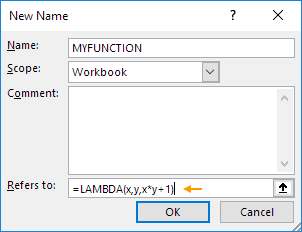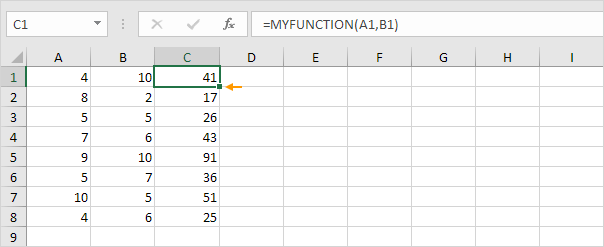# LAMBDA

Use LAMBDA and the Name Manager in Excel to create your own Excel function. You can use this custom function like any other Excel function!

Suppose we want to create a custom function called MYFUNCTION that multiplies two values, and then adds 1 to the result.To create this function, execute the following steps.

1. On the Formulas tab, in the Defined Names group, click Define Name.2. Give your function a name.3. Use LAMBDA to create your function.Explanation: first specify the input arguments (x and y). Finish with a calculation to define the output of the function (x*y+1).

4. A LAMBDA function works like any other Excel function. For example, double click the lower right corner of cell C1 to quickly copy this function to the other cells.Note: if you like, you can use different argument names. In this example, =LAMBDA(a,b,a*b+1) produces the exact same result! The LAMBDA function is only available in Excel 365 or Excel 2021.

Go to Next Chapter: Sort